JEE  >  JEE Main Mock - 6 (Previous Pattern)

# JEE Main Mock - 6 (Previous Pattern)

Test Description

## 90 Questions MCQ Test Mock Test Series for JEE Main & Advanced | JEE Main Mock - 6 (Previous Pattern)

JEE Main Mock - 6 (Previous Pattern) for JEE 2022 is part of Mock Test Series for JEE Main & Advanced preparation. The JEE Main Mock - 6 (Previous Pattern) questions and answers have been prepared according to the JEE exam syllabus.The JEE Main Mock - 6 (Previous Pattern) MCQs are made for JEE 2022 Exam. Find important definitions, questions, notes, meanings, examples, exercises, MCQs and online tests for JEE Main Mock - 6 (Previous Pattern) below.
Solutions of JEE Main Mock - 6 (Previous Pattern) questions in English are available as part of our Mock Test Series for JEE Main & Advanced for JEE & JEE Main Mock - 6 (Previous Pattern) solutions in Hindi for Mock Test Series for JEE Main & Advanced course. Download more important topics, notes, lectures and mock test series for JEE Exam by signing up for free. Attempt JEE Main Mock - 6 (Previous Pattern) | 90 questions in 180 minutes | Mock test for JEE preparation | Free important questions MCQ to study Mock Test Series for JEE Main & Advanced for JEE Exam | Download free PDF with solutions
 1 Crore+ students have signed up on EduRev. Have you?
JEE Main Mock - 6 (Previous Pattern) - Question 1

### A d.c. motor having an armature of resistance 2 Ω is designed to operate at 220 V mains. At full speed, it develops a back e.m.f. of 210 V. When the motor is running at full speed, the current in the armature is

Detailed Solution for JEE Main Mock - 6 (Previous Pattern) - Question 1

When motor is running at full speed the electric current is given by: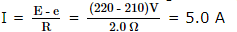JEE Main Mock - 6 (Previous Pattern) - Question 2

### A solenoid has an inductance of 60 henrys and a resistance of 30 ohms. If it is connected to a 100 volt battery, how long will it take for the current to reach e-1/e=63.2% of its final value

JEE Main Mock - 6 (Previous Pattern) - Question 3

### A dipole of dipole moment p̅ is placed in uniform electric field E̅, then torque acting on it is given by

JEE Main Mock - 6 (Previous Pattern) - Question 4

What fraction of a radioactive material will get disintegrated in a period of two half-lives

Detailed Solution for JEE Main Mock - 6 (Previous Pattern) - Question 4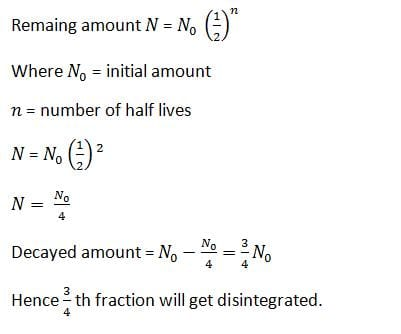JEE Main Mock - 6 (Previous Pattern) - Question 5

What percentage of original radioactive atoms is left after five half lives ?

JEE Main Mock - 6 (Previous Pattern) - Question 6

Photons of energy 5.5eV fall on the surface of the metal emitting photoelectrons of maximum kinetic energy 4.0eV. The stopping potential required for these electrons are :

JEE Main Mock - 6 (Previous Pattern) - Question 7

The angular speed of an engine wheel making 90 revolutions per minute is

JEE Main Mock - 6 (Previous Pattern) - Question 8

An electric dipole is placed along the X-axis at the origin O. A point P is at a distance of 20 cm from this origin such that OP makes an angle π/3 with the X axis. If electric field at P makes an angle θ with X-axis, the value of θ is

JEE Main Mock - 6 (Previous Pattern) - Question 9

A man of mass 60 kg. records his wt. on a weighing machine placed inside a lift. The ratio of wts. of man recorded when lift is ascending up with a uniform speed of 2 m/s to when it is descending down with a uniform speed of 4 m/s will be

JEE Main Mock - 6 (Previous Pattern) - Question 10

A boat of mass 40 kg is at rest. If a dog of mass 4 kg moves in the boat with a velocity of 10 m/s, then velocity of a boat will be

Detailed Solution for JEE Main Mock - 6 (Previous Pattern) - Question 10

According to law of conservation of momentum
(md + mbb = md υd
⇒ 44 υb = 4 x 10
⇒ υb = 1 m/s

JEE Main Mock - 6 (Previous Pattern) - Question 11

A microammeter has a resistance of 100 Ω and full scale range of 50 μ A. It can be used as a voltmeter or as a higher range ammeter provided a resistance is added to it. Pick the correct range and resistance combination

JEE Main Mock - 6 (Previous Pattern) - Question 12

Spherical balls of radius R are falling in a viscous fluid of viscosity η with a velocity v. The retarding viscous force acting on the spherical ball is

JEE Main Mock - 6 (Previous Pattern) - Question 13

The symbolic representation of four logic gates are given below: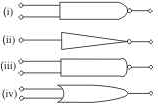The logic symbols for OR, NOT and NAND gates are respectively:

JEE Main Mock - 6 (Previous Pattern) - Question 14

The size of nucleus of an atom of mass number A is proportional to

JEE Main Mock - 6 (Previous Pattern) - Question 15

A ball is moving to and fro about the lowest point A of a smooth hemisphrical bowl. If it is able to rise up to a height of 20 cm on either side of A, its speed at A must be (Take = 10 m/s2. mass of the body 5 g)

JEE Main Mock - 6 (Previous Pattern) - Question 16

In the following question, a Statement of Assertion (A) is given followed by a corresponding Reason (R) just below it. Read the Statements carefully and mark the correct answer-
Assertion(A): By the study of positive rays, Thomson discovered the existence of isotopes.
Reason(R): Thomson obtained two parabolas for neon gas whose atomic mass is 20.2 .

JEE Main Mock - 6 (Previous Pattern) - Question 17

In the following question, a Statement of Assertion (A) is given followed by a corresponding Reason (R) just below it. Read the Statements carefully and mark the correct answer-
Assertion(A): When a massive projectile collides with a lighter stationary target then maximum speed of target is twice that of projectile.
Reason(R): It is explained by the momentum and energy conservation.

JEE Main Mock - 6 (Previous Pattern) - Question 18

Two rings of the same radius and mass are placed such that their centres are at a common point and their planes are perpendicular to each other. The moment of inertia of the system about an axis passing through the centre and perpendicular to the plane of one of the ring is (mass = and radius = r)

JEE Main Mock - 6 (Previous Pattern) - Question 19

A process in which volume remains constant is called

JEE Main Mock - 6 (Previous Pattern) - Question 20

Erg-m⁻1 can be the unit of measure for

JEE Main Mock - 6 (Previous Pattern) - Question 21
In Young's experiment of double slit, the intensity of central bright band is ---- times the individual intensities of the interfering waves
JEE Main Mock - 6 (Previous Pattern) - Question 22
A train is approaching a platform with a velocity ν. The frequency heard by an observer standing at the station is
JEE Main Mock - 6 (Previous Pattern) - Question 23
To demonstrate the phenomenon of interference we require two sources which emit radiations of
JEE Main Mock - 6 (Previous Pattern) - Question 24
Standing stationary waves can be obtained in an air column even if the interfering waves are
JEE Main Mock - 6 (Previous Pattern) - Question 25

The H α line is the first member of the Balmer series of the hydrogen spectrum and it occurs due to the transition of the electron from the 3rd orbit to the 2ndorbit. If its wave length is λ , the wave length of the last member of the Balmer series will be

JEE Main Mock - 6 (Previous Pattern) - Question 26

If the charge on a capacitor is increased by 2 coulomb, the energy stored in it increases by 21%. The original charge on the capacitor is

JEE Main Mock - 6 (Previous Pattern) - Question 27

A particle is moving on a circular path with constant speed, then its acceleration will be

JEE Main Mock - 6 (Previous Pattern) - Question 28

At what temperature will the resistance of a copper wire be three times its value at 0ºC? (Given Temperature coefficient of resistance for copper = 4x10⁻3ºC⁻1)

Detailed Solution for JEE Main Mock - 6 (Previous Pattern) - Question 28 For temp. coeff (a)=4×10^-3,
3R=R(1+(a)t)
2/4×10^-3=t
t=500
JEE Main Mock - 6 (Previous Pattern) - Question 29

How many electrons must be removed from each of two 5.0-kg copper spheres to make the electric force of repulsion between them equal in magnitude to the gravitational attraction between them?

JEE Main Mock - 6 (Previous Pattern) - Question 30

There are three voltmeters of the same range but of resistance 10000 Ω, 8000 Ω and 4000 Ω respectively. The best voltmeter among these is the one whose resistance is

JEE Main Mock - 6 (Previous Pattern) - Question 31

Final product formed on reduction of glycerol by hydriodic acid is

JEE Main Mock - 6 (Previous Pattern) - Question 32

RCH = O + H₂ → RCH₂OH. For this reaction, catalyst is

JEE Main Mock - 6 (Previous Pattern) - Question 33

Night blindness is caused by the deficiency of

JEE Main Mock - 6 (Previous Pattern) - Question 34

For the equilibrium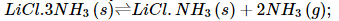K p = 9 a t m 2 at 37 º C . A 5 litre vessel contains 0.1 mole of LiCl.NH3. How many moles of NH3 should be added to the flask at this temperature to derive the backward reaction for completion?
Use R = 0.082 atm -L/mol K

JEE Main Mock - 6 (Previous Pattern) - Question 35

If initial concentration is reduced to (1/4)th in a zero order reaction, the time taken for half the reaction to complete

JEE Main Mock - 6 (Previous Pattern) - Question 36

Given that heat of neutralisation of strong acid and strong base is -57.1 kJ. Calculate the heat produced when 0.25 mole of HCl is neutralised with 0.25 mole of NaOH in aqueous solution.

Detailed Solution for JEE Main Mock - 6 (Previous Pattern) - Question 36

The heat produced when 0.25 mole of HCl is neutralised with 0.25 mole of NaOH in aqueous solution is 57.1x1/4=14.275kJ

JEE Main Mock - 6 (Previous Pattern) - Question 37

The decreasing order of the ionisation potential of the following elements is

Detailed Solution for JEE Main Mock - 6 (Previous Pattern) - Question 37

As we move across a period from left to right, the nuclear charge increases and the atomic radius decreases though the principal quantum number of the valence shell remains the same. As a result, the valence electrons are more are more tightly held by the nucleus, hence ionisation potential keep on increasing. However, closed shell configuration (Ne), half-filled configuration (P) and completely-filled configuration (Mg) are responsible for higher values of ionisation energy.

JEE Main Mock - 6 (Previous Pattern) - Question 38

In the complex compound K₄[Ni(CN)₄], the oxidation no. of nickel is

JEE Main Mock - 6 (Previous Pattern) - Question 39

Which of the following reagent can be used to convert benzene diazonium chloride into benzene ?

JEE Main Mock - 6 (Previous Pattern) - Question 40

The element, which forms oxides in all oxidation states + 1 to + 5, is

JEE Main Mock - 6 (Previous Pattern) - Question 41

The e.m.f. of a galvanic cell with electrode potentials of silver equal to + 0.80 V and that of copper equal to + 0.34 V is

JEE Main Mock - 6 (Previous Pattern) - Question 42

Which of the following compound is the most soluble in water?

JEE Main Mock - 6 (Previous Pattern) - Question 43

The best indicator for the detection of end point in titration of a strong base is

JEE Main Mock - 6 (Previous Pattern) - Question 44

The pH indicates are

JEE Main Mock - 6 (Previous Pattern) - Question 45

The pH of blood does not appreciably change by a small addition of acid or a base because blood

JEE Main Mock - 6 (Previous Pattern) - Question 46

Which of the following does not give yellow ppt. with I₂/NaOH?

JEE Main Mock - 6 (Previous Pattern) - Question 47

Product(s) of the following S N 1 reaction is/are :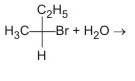JEE Main Mock - 6 (Previous Pattern) - Question 48

An example of natural biopolymer is

JEE Main Mock - 6 (Previous Pattern) - Question 49

Which of the following isomers will have the highest octane number ?

JEE Main Mock - 6 (Previous Pattern) - Question 50

Which of the following metal oxides is antiferromagnetic in nature?

JEE Main Mock - 6 (Previous Pattern) - Question 51

The radius of an atom of an element is 500 pm, if it crystallizes as a face-centred cubic lattice, then the edge length of the unit cell is

JEE Main Mock - 6 (Previous Pattern) - Question 52

The normality of orthophosphoric acid having purity of 70% by weight and specific gravity 1.54 would be (H=1;O=16; P=31)

JEE Main Mock - 6 (Previous Pattern) - Question 53

A solution contains non-volatile solute of molecular mass M₂. which of the following can be used to calculate the molecules mass of solute in terms of osmotic pressure ?

JEE Main Mock - 6 (Previous Pattern) - Question 54

Catalyst used in dimerisation of acetylene to prepare chloroprene is

JEE Main Mock - 6 (Previous Pattern) - Question 55

The ionisation potential of hydrogen atom is 13.6 eV. The energy required to remove an electron in the n = 2 state of the hydrogen atom is __

Detailed Solution for JEE Main Mock - 6 (Previous Pattern) - Question 55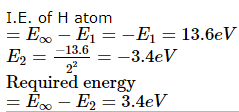JEE Main Mock - 6 (Previous Pattern) - Question 56

The electronic configuration of the atom with atomic number 47 is ___

Detailed Solution for JEE Main Mock - 6 (Previous Pattern) - Question 56

Element with atomic number 47 is silver (Ag) with configuration (actual is 1s2 22 2p6 3s2 3p6 3d10 4s2 4p6 4d10 5s1, i.e. its shows preference for completely filled orbital.

JEE Main Mock - 6 (Previous Pattern) - Question 57

The change in entropy ∆S is positive for certain reaction and enthalpy change ∆H is also greater than zero at temperature T.The reaction is

JEE Main Mock - 6 (Previous Pattern) - Question 58

The reaction of acidified, aqueous potassium iodide with aqueous hydrogen peroxide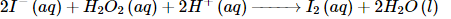is thought to involve the following steps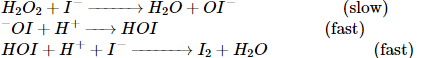Which one of the following conclusions cannot be drawn from this information?

JEE Main Mock - 6 (Previous Pattern) - Question 59

Which of the following sequence follows the Irwing-William order?

Detailed Solution for JEE Main Mock - 6 (Previous Pattern) - Question 59

The correct order is according to decreasing charge to radius ratio.

JEE Main Mock - 6 (Previous Pattern) - Question 60

Which of the following compounds to does not show Lassaigne's test for nitrogen?

JEE Main Mock - 6 (Previous Pattern) - Question 61

If (a2)+16(b2)+49(c2)-4ab-28bc-7ac=0, then a,b,c are in:

JEE Main Mock - 6 (Previous Pattern) - Question 62

If 2 x + 3 y = 17 and 2x + 2 − 3 y + 1 = 5, then what is the value of x ?

JEE Main Mock - 6 (Previous Pattern) - Question 63

AOB is the positive quadrant of the ellipse x 2 ∕ a 2 + y 2 ∕ b 2 = 1, where OA = a, OB = b. The area between the arc AB and the chord AB of the ellipse is

JEE Main Mock - 6 (Previous Pattern) - Question 64

The angle between the tangents drawn from (0,0) to the circle x2 + y2 + 4x - 6y + 4 = 0 is

JEE Main Mock - 6 (Previous Pattern) - Question 65

If 2x - 3y = 0 is the equation of the common chord of the circles x2 + y2 + 4x = 0 and x2 + y2 + 2λy = 0, then the value of λ is

JEE Main Mock - 6 (Previous Pattern) - Question 66

If the cube roots of unity are 1,ω,ω2 then the roots of the equation (x - 1)3 + 8 = 0,are

JEE Main Mock - 6 (Previous Pattern) - Question 67

The differential equation obtained by eliminating the arbitrary constants a and b from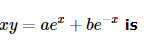JEE Main Mock - 6 (Previous Pattern) - Question 68

At x=3, (d/dx)[|x-1|+|x-5|]=

JEE Main Mock - 6 (Previous Pattern) - Question 69

Let f(x) = x, g(x) = 1/x and h(x) = f(x)g(x). Then h(x) = 1 if

JEE Main Mock - 6 (Previous Pattern) - Question 70

Which is logically equivalent to "If today is Sunday, Matt cannot play hockey."?

Detailed Solution for JEE Main Mock - 6 (Previous Pattern) - Question 70

Statements are logically equivalent to their contrapositives.

JEE Main Mock - 6 (Previous Pattern) - Question 71

In the following question, a Statement of Assertion (A) is given followed by a corresponding Reason (R) just below it. Read the Statements carefully and mark the correct answer-
Assertion(A): Sum of the last 30 coefficients in the expansion of (1+x)59, when expanded in ascending powers of x, is 258.
Reason(R): pth term from the end in the expansion of (x+y)n is (n - p + 2)th term from the beginning.

JEE Main Mock - 6 (Previous Pattern) - Question 72

In the following question, a Statement of Assertion (A) is given followed by a corresponding Reason (R) just below it. Read the Statements carefully and mark the correct answer-
Assertion(A): The number of terms in the expansion of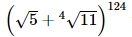which are integers is equal to 32.
Reason(R): In the binomial expansion of (x+y)n , the (r+1)th term from the beginning is called general term (Tr+1) i.e, Tr+1 = nCr xn+ryr.

Detailed Solution for JEE Main Mock - 6 (Previous Pattern) - Question 72 The correct option is B.
Using Binomial approximation every 4 th term and the first term is an integer
hence no. of integral values=1+(124/4)=1+31=32
this is not explained by the reason
JEE Main Mock - 6 (Previous Pattern) - Question 73

If nP4:nP3=1:2, then n=

JEE Main Mock - 6 (Previous Pattern) - Question 74

The line, among the following, that touches the parabola y2 = 4ax is

JEE Main Mock - 6 (Previous Pattern) - Question 75

Each coefficients in the equation ax2+bx+c=0 is determined by throwing an ordinary die. The probability that the equation will have equal roots is

JEE Main Mock - 6 (Previous Pattern) - Question 76

If the two lines of regression of a bivariate distribution coincide, then the correlation coefficient ρ satisfies

JEE Main Mock - 6 (Previous Pattern) - Question 77

The foot of perpendicular from (2,4) on the line x+y=1 is

JEE Main Mock - 6 (Previous Pattern) - Question 78

The length of the subnormal to the curve y2 = 2px is

JEE Main Mock - 6 (Previous Pattern) - Question 79

Set of equations a+b-2c = 0, 2a-3b+c = 0 and a-5b+4c = α is consistent, if α equal to

JEE Main Mock - 6 (Previous Pattern) - Question 80

If 1, ω, ω2 are cube roots of unity, the root of the equation (x-1)3 + 8 = 0 are

JEE Main Mock - 6 (Previous Pattern) - Question 81

If cot (α+β)=0, then sin (α+2β) is equal to

JEE Main Mock - 6 (Previous Pattern) - Question 82

The angle between the planes 2x - y + z = 6 and x + y + 2z = 7 is

JEE Main Mock - 6 (Previous Pattern) - Question 83

The area of a parallelogram whose adjacent sides are a=i+2j+3k, b=3i-2j+k, is

JEE Main Mock - 6 (Previous Pattern) - Question 84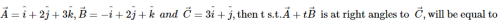Detailed Solution for JEE Main Mock - 6 (Previous Pattern) - Question 84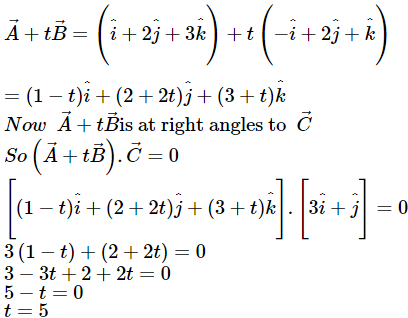JEE Main Mock - 6 (Previous Pattern) - Question 85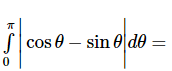JEE Main Mock - 6 (Previous Pattern) - Question 86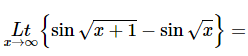JEE Main Mock - 6 (Previous Pattern) - Question 87

Suppose a,b,c are in A.P. and a2,b2,c2 are in G.P. If a < b< c and a + b + c =3/2,then the value of a is

JEE Main Mock - 6 (Previous Pattern) - Question 88

In the following question, a Statement of Assertion (A) is given followed by a corresponding Reason (R) just below it. Read the Statements carefully and mark the correct answer-
Assertion(A): The number of ways of selecting two elements x and y from the set S = {1, 5, 9, 13,...,1093} such that x + y = 1094 is 137.
Reason(R) : The elements of S are in A.P. with common difference 4 and number of terms 274.

JEE Main Mock - 6 (Previous Pattern) - Question 89

If A and B are matrices such that A+B and AB are defined, then

JEE Main Mock - 6 (Previous Pattern) - Question 90

The maximum value of x3 - 3x in [0,2] is

## Mock Test Series for JEE Main & Advanced

2 videos|324 docs|160 tests
 Use Code STAYHOME200 and get INR 200 additional OFF Use Coupon Code
Information about JEE Main Mock - 6 (Previous Pattern) Page
In this test you can find the Exam questions for JEE Main Mock - 6 (Previous Pattern) solved & explained in the simplest way possible. Besides giving Questions and answers for JEE Main Mock - 6 (Previous Pattern), EduRev gives you an ample number of Online tests for practice

## Mock Test Series for JEE Main & Advanced

2 videos|324 docs|160 tests